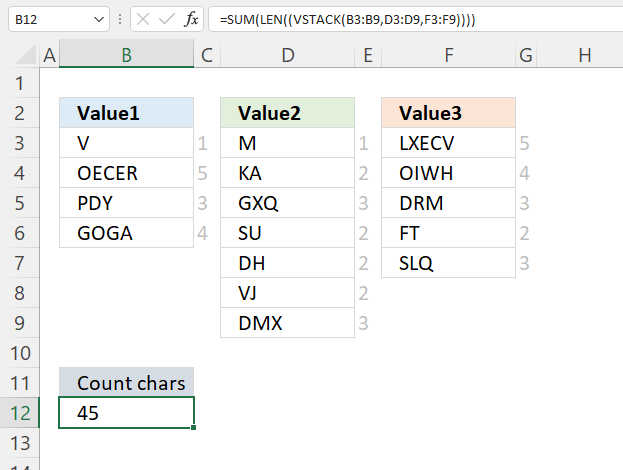Author: Oscar Cronquist Article last updated on June 02, 2022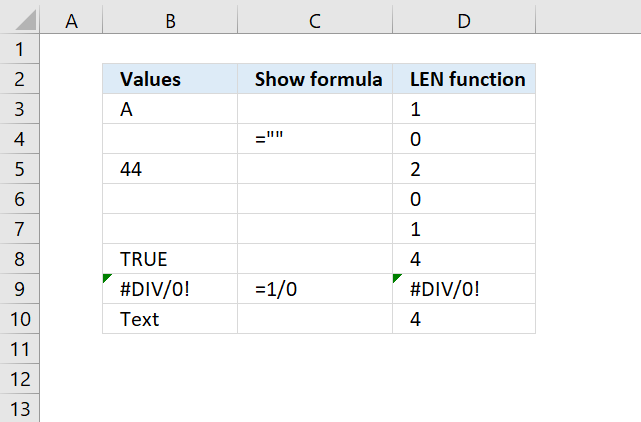The LEN function returns the number of characters in a cell value.

LEN(text)

## 2. LEN Function Arguments

 text Required. The text string or cell reference you want to count the number of characters in.

The LEN function does not count the number of characters in a formula, however, it counts the number of characters a formula returns.

## 3. LEN Function Example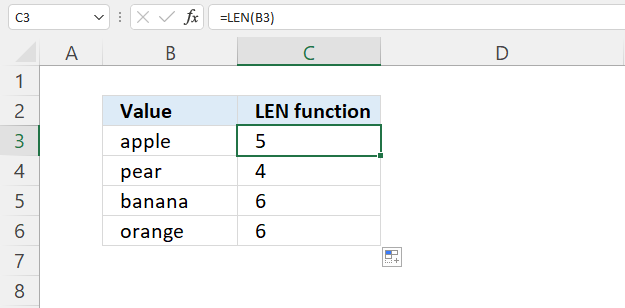Formula in cell C3:

=LEN(B3)

The LEN function counts all characters in a given cell. You can also use a cell range, however, the output is an array of values. You need to enter the formula as an array formula if you use an Excel version earlier than Excel 365, as far as I know.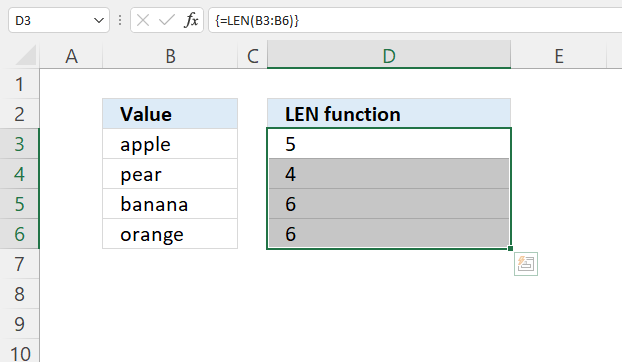Array formula in cell D3:D6:

=LEN(B3:B6)

Excel 365 subscribers may enter the formula as a regular formula, the values spill automatically to cells below as far as needed.

## 4. Count characters based on condition example 1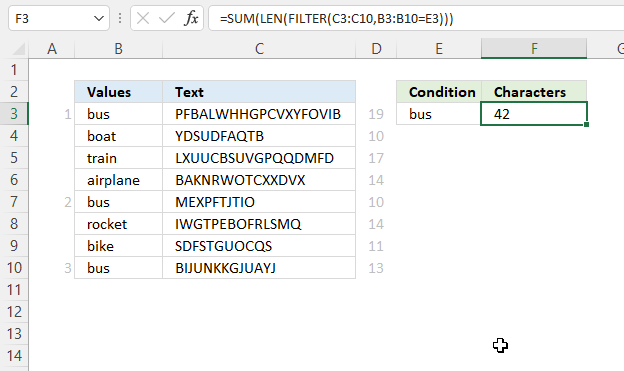Excel 365 dynamic array formula in cell F3:

=SUM(LEN(FILTER(C3:C10, B3:B10=E3)))

### 4.1 Explaining formula

#### Step 1 - Logical expression

The equal sign lets you compare value to value, you can also compare value to an array of values. The result is an array of boolean values TRUE or FALSE with the same number of items as the original array.

B3:B10=E3

becomes

{"bus"; "boat"; "train"; "airplane"; "bus"; "rocket"; "bike"; "bus"}="bus"

and returns

{TRUE; FALSE; FALSE; FALSE; TRUE; FALSE; FALSE; TRUE}.

#### Step 2 - Filter values based on a logical expression

The FILTER function lets you extract values/rows based on a condition or criteria.
FILTER(arrayinclude, [if_empty])

FILTER(C3:C10, B3:B10=E3)

becomes

FILTER({"PFBALWHHGPCVXYFOVIB"; "YDSUDFAQTB"; "LXUUCBSUVGPQQDMFD"; "BAKNRWOTCXXDVX"; "MEXPFTJTIO"; "IWGTPEBOFRLSMQ"; "SDFSTGUOCQS"; "BIJUNKKGJUAYJ"}, {TRUE; FALSE; FALSE; FALSE; TRUE; FALSE; FALSE; TRUE})

and returns

{"PFBALWHHGPCVXYFOVIB"; "MEXPFTJTIO"; "BIJUNKKGJUAYJ"}.

#### Step 3 - Count characters in the array, value by value

LEN(FILTER(C3:C10, B3:B10=E3))

becomes

LEN({"PFBALWHHGPCVXYFOVIB"; "MEXPFTJTIO"; "BIJUNKKGJUAYJ"})

and returns

{19; 10; 13}.

#### Step 4 - Add numbers and return a total

The SUM function calculates a total.
SUM(number1, [number2], ...)

SUM(LEN(FILTER(C3:C10, B3:B10=E3)))

becomes

SUM({19; 10; 13})

and returns 42.

## 5. Count characters based on condition example 2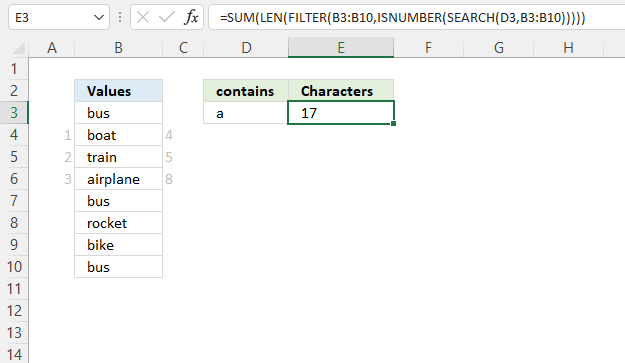Excel 365 dynamic array formula in cell E3:

=SUM(LEN(FILTER(B3:B10,ISNUMBER(SEARCH(D3,B3:B10)))))

### 5.1 Explaining formula

#### Step 1 - Find string in cell range B3:B10

The SEARCH function returns a number representing the position of the character at which a specific text string is found reading left to right.
SEARCH(find_text,within_text, [start_num])

SEARCH(D3, B3:B10)

becomes

SEARCH("a", {"bus"; "boat"; "train"; "airplane"; "bus"; "rocket"; "bike"; "bus"})

and returns

{#VALUE!; 3; 3; 1; #VALUE!; #VALUE!; #VALUE!; #VALUE!}

#### Step 2 - Check if number

The ISNUMBER function checks if a value is a number, and returns TRUE or FALSE.
ISNUMBER(value)

ISNUMBER(SEARCH(D3,B3:B10))

becomes

ISNUMBER({#VALUE!; 3; 3; 1; #VALUE!; #VALUE!; #VALUE!; #VALUE!})

and returns

{FALSE; TRUE; TRUE; TRUE; FALSE; FALSE; FALSE; FALSE}.

#### Step 3 - Filter values

The FILTER function lets you extract values/rows based on a condition or criteria.
FILTER(arrayinclude, [if_empty])

FILTER(B3:B10,ISNUMBER(SEARCH(D3,B3:B10)))

becomes

FILTER(B3:B10,{FALSE; TRUE; TRUE; TRUE; FALSE; FALSE; FALSE; FALSE})

and returns

{"boat"; "train"; "airplane"}.

#### Step 4 - Count characters

LEN(FILTER(B3:B10,ISNUMBER(SEARCH(D3,B3:B10))))

becomes

LEN({"boat"; "train"; "airplane"})

and returns

{4; 5; 8}.

#### Step 5 - Add numbers and return a total

The SUM function calculates a total.
SUM(number1, [number2], ...)

SUM(LEN(FILTER(B3:B10, ISNUMBER(SEARCH(D3, B3:B10)))))

becomes

SUM({4; 5; 8})

and returns 17.

## 6. Count characters based on a list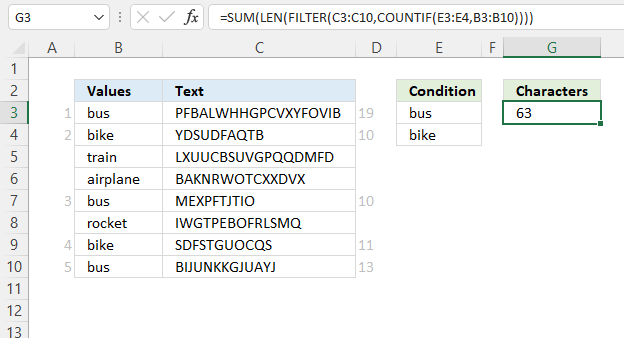Excel 365 dynamic array formula in cell F3:

=SUM(LEN(FILTER(C3:C10, COUNTIF(E3:E4,B3:B10))))

### 6.1 Explaining formula

#### Step 1 - Compare the list with values in B3:B10

The COUNTIF function calculates the number of cells that meet a given condition.
COUNTIF(rangecriteria)

COUNTIF(E3:E4,B3:B10)

becomes

COUNTIF({"bus"; "bike"},{"bus"; "bike"; "train"; "airplane"; "bus"; "rocket"; "bike"; "bus"})

and returns

{1; 1; 0; 0; 1; 0; 1; 1}.

#### Step 2 - Filter values based on a logical expression

The FILTER function lets you extract values/rows based on a condition or criteria.
FILTER(arrayinclude, [if_empty])

FILTER(C3:C10, COUNTIF(E3:E4,B3:B10))

becomes

FILTER({"PFBALWHHGPCVXYFOVIB"; "YDSUDFAQTB"; "LXUUCBSUVGPQQDMFD"; "BAKNRWOTCXXDVX"; "MEXPFTJTIO"; "IWGTPEBOFRLSMQ"; "SDFSTGUOCQS"; "BIJUNKKGJUAYJ"}, {1; 1; 0; 0; 1; 0; 1; 1})

and returns

{"PFBALWHHGPCVXYFOVIB"; "YDSUDFAQTB"; "MEXPFTJTIO"; "SDFSTGUOCQS"; "BIJUNKKGJUAYJ"}.

#### Step 3 - Count characters in the array, value by value

LEN(FILTER(C3:C10, COUNTIF(E3:E4,B3:B10)))

becomes

LEN({"PFBALWHHGPCVXYFOVIB"; "YDSUDFAQTB"; "MEXPFTJTIO"; "SDFSTGUOCQS"; "BIJUNKKGJUAYJ"})

and returns

{19; 10; 10; 11; 13}.

#### Step 4 - Add numbers and return a total

The SUM function calculates a total.
SUM(number1, [number2], ...)

SUM(LEN(FILTER(C3:C10, COUNTIF(E3:E4,B3:B10))))

becomes

SUM({19; 10; 10; 11; 13})

and returns 63. 19 + 10 + 10 + 11 + 13 equals 63.

## 7. Count characters in a formula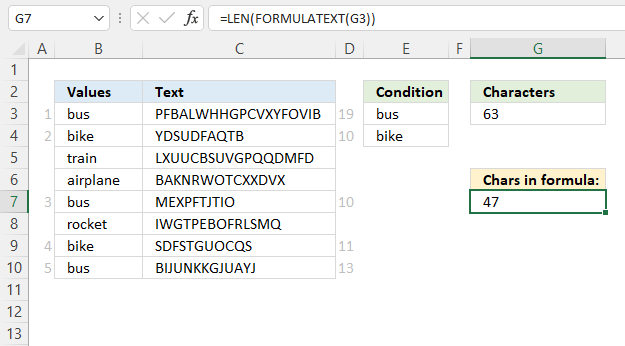Formula in cell G7:

=LEN(FORMULATEXT(G3))

### 7.1 Explaining formula

#### Step 1 - Extract formula text

The FORMULATEXT function returns the contents of a cell as long as it contains a formula.
FORMULATEXT(reference)

FORMULATEXT(G3)

returns

"=SUM(LEN(FILTER(C3:C10,COUNTIF(E3:E4,B3:B10))))"

#### Step 2 - Count characters

LEN(FORMULATEXT(G3))

becomes

LEN("=SUM(LEN(FILTER(C3:C10,COUNTIF(E3:E4,B3:B10))))")

and returns 47.

## 8. Count characters in multiple cell ranges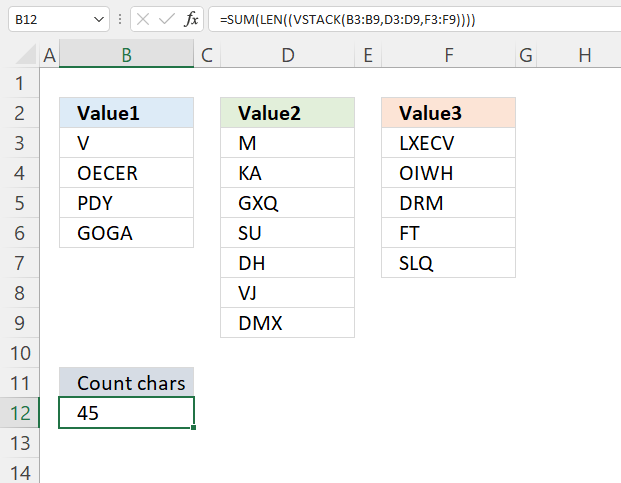Formula in cell B12:

=SUM(LEN((VSTACK(B3:B9,D3:D9,F3:F9))))

### 7.1 Explaining formula

#### Step 1 - Join cell ranges vertically

The VSTACK function lets you join cell ranges, it joins data to the first blank cell at the bottom of a cell range or array.
VSTACK(array1,[array2],...)

VSTACK(B3:B9,D3:D9,F3:F9)

becomes

VSTACK({"V"; "OECER"; "PDY"; "GOGA"; 0; 0; 0},{"M"; "KA"; "GXQ"; "SU"; "DH"; "VJ"; "DMX"},{"LXECV"; "OIWH"; "DRM"; "FT"; "SLQ"; 0; 0})

and returns

{"V"; "OECER"; "PDY"; "GOGA"; 0; 0; 0; "M"; "KA"; "GXQ"; "SU"; "DH"; "VJ"; "DMX"; "LXECV"; "OIWH"; "DRM"; "FT"; "SLQ"; 0; 0}

#### Step 2 - Count characters

LEN((VSTACK(B3:B9,D3:D9,F3:F9))

becomes

LEN({"V"; "OECER"; "PDY"; "GOGA"; 0; 0; 0; "M"; "KA"; "GXQ"; "SU"; "DH"; "VJ"; "DMX"; "LXECV"; "OIWH"; "DRM"; "FT"; "SLQ"; 0; 0})

and returns

{1; 5; 3; 4; 0; 0; 0; 1; 2; 3; 2; 2; 2; 3; 5; 4; 3; 2; 3; 0; 0}.

#### Step 3 - Add numbers and return a total

The SUM function calculates a total.
SUM(number1, [number2], ...)

SUM(LEN((VSTACK(B3:B9,D3:D9,F3:F9))))

becomes

SUM({1; 5; 3; 4; 0; 0; 0; 1; 2; 3; 2; 2; 2; 3; 5; 4; 3; 2; 3; 0; 0})

and returns 45.